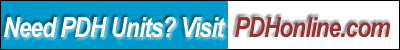Print this page

Introduction to Analysis of Variance (ANOVA)

David J. Lilja, Ph.D., P.E.

Course Overview

Analysis of variance (ANOVA) is a general technique for separating the total variation in a set of measurements into the variation due to measurement noise and the variation due to real differences among the alternatives being compared. This course provides a gentle introduction to comparing a set of alternatives using the ANOVA technique.

This course includes a multiple-choice quiz at the end, which is designed to enhance the understanding of the course materials.

Learning Objective

After completing this 3-hour course, you will be able to:

• Use the ANOVA technique to determine whether there is a statistically significant difference in the performance of k different systems;
• Compute the effects of the different alternatives;
• Compute the mean square values of the effects and the alternatives;
• Use the F-test to determine if two variances are statistically different; and
• Use contrasts to determine where the differences in the alternatives occur.

The reading assignment for this course is Chapter 5.2 of Measuring Computer Performance: A Practitioner's Guide, David J. Lilja, Cambridge University Press, 2000.

If you don't have this book, you can purchase Chapter 1 in PDF format online at eBooks.com for a modest cost. The price for this course listed on this website does not include the cost of purchasing the chapter through eBooks.com. However, the price has been reduced to compensate for the cost of purchasing the chapter required. If you plan to take all 6 courses (E132 to E137) based on this book, you may consider to purchase a hard copy of the book or the entire book in PDF format online through eBooks.com.

Key Terms

• ANOVA
• Analysis of Variance
• One-factor experiment
• Variation
• Effects
• Mean square value
• F-test
• Contrasts

Study Notes

Consider a situation in which you are trying to compare k different computer systems. You make n measurements of the execution time of a benchmark program on each of the systems for a total of kn unique measurements. Due to measurement noise, it is likely that none of the measurements will be the same. However, it appears that there could be some differences between the systems in spite of the noise in your measurements. How can you sort through all of these measurements to determine whether there actually are real differences between the systems, or whether the differences you see are due simply to measurement noise (errors)?

Analysis of variance (ANOVA) is a very general statistical technique developed precisely for sorting through these types of measurement experiments.

The basic idea behind ANOVA is to begin by determining the total variation observed in all of the measurements. This variation then is partitioned into two components. The first component is the variation within a single system. This variation is assumed to be caused by measurement noise only. The second component is the variation in the measured values between the systems being compared. This second component of the variation is due to both measurement error and, potentially, due to real differences between the systems.

ANOVA provides us with a technique for comparing these two components of the variation in all of the measurements to determine if the variation between systems is statistically larger than the variation due to the measurement noise within a system. If the variation due to actual differences among the alternatives is enough larger than the variation due to measurement noise, then we can say that there is a statistically significant difference in the performance of the systems tested. The key is determining how much is "enough larger" to be statistically significant.

The ANOVA technique can be extended to more than one input (factor), as discussed in Chapter 9 of the course text. However, the basic idea as described in this course remains the same.

Quiz

Once you finish studying the above course content, you need to take a quiz to obtain the PDH credits.

DISCLAIMER: The materials contained in the online course are not intended as a representation or warranty on the part of PDH Center or any other person/organization named herein. The materials are for general information only. They are not a substitute for competent professional advice. Application of this information to a specific project should be reviewed by a registered architect and/or professional engineer/surveyor. Anyone making use of the information set forth herein does so at their own risk and assumes any and all resulting liability arising therefrom.